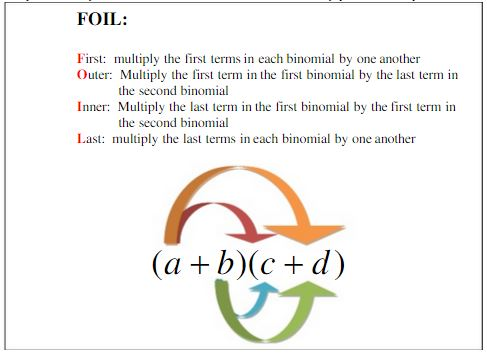Ontario Tech acknowledges the lands and people of the Mississaugas of Scugog Island First Nation.

We are thankful to be welcome on these lands in friendship. The lands we are situated on are covered by the Williams Treaties and are the traditional territory of the Mississaugas, a branch of the greater Anishinaabeg Nation, including Algonquin, Ojibway, Odawa and Pottawatomi. These lands remain home to many Indigenous nations and peoples.

We acknowledge this land out of respect for the Indigenous nations who have cared for Turtle Island, also called North America, from before the arrival of settler peoples until this day. Most importantly, we acknowledge that the history of these lands has been tainted by poor treatment and a lack of friendship with the First Nations who call them home.

This history is something we are all affected by because we are all treaty people in Canada. We all have a shared history to reflect on, and each of us is affected by this history in different ways. Our past defines our present, but if we move forward as friends and allies, then it does not have to define our future.

# Expanding

## A Monomial Times a Binomial

If we have a monomial (the product of powers of variables) multiplying a binomial (2 monomials added together), then we simply make sure that the monomial multiplies both terms in the binomial.

Example: Expand 5x2 (3x -1)

Solution:

5x2 (3x -1)

=(5x2)(3x)+(5x2)(-1)

=15x3 - 5x2

## A Binomial Times a Binomial

To multiply 2 binomials together, we need to make sure that each term in each binomial gets multiplied by each term in the other binomial. To help us do this, there is a useful acronym that helps us remember which terms we must multiply. The acronym is:Example: Expand (x-7)(x+1)

Solution:

(x-7)(x+1)

=(x)(x)+(x)(1)+(-7)(x)+(-7)(1)

=x2+x-7x-7

=x2 -6x -7

Example: Expand (3x + 2)(2x - 5).

Solution:

(3x + 2)(2x - 5)

=(3x)(2x)+(3x)(-5)+(2)(2x)+(2)(-5)

=6x- 15x + 4x - 10

=6x2 -11x -10

A Few Notes

• If you have to expand the expressions you're expanding contain more than 2 terms each, just apply the principle that each term in the first expression must multiply each term in the second expression...that's the general rule that the FOIL idea was based on.
• If you have to multiply more than two expressions together, just proceed two at a time.
• If you know how to factor, remember that expanding is the opposite process of factoring, so if you were to factor after you're done expanding, you'd get the original expression in the question. Similarly, if you're to expand after factoring you'd get the original expression as well...this is a useful way to check your work!

Example 1:

Example 2:

Example 3:

Avoiding Common Math Mistakes when Expanding: